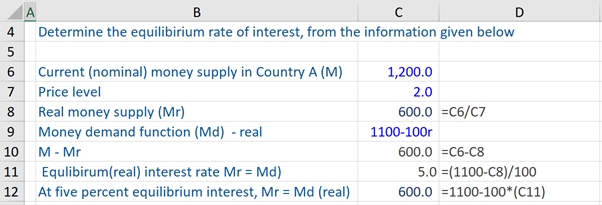## What is “Determination of Interest Rates”?

The interest rates in an economy are determined by the forces of demand and supply of money. Therefore, the determination of interest rates depends on factors that influence both demand for and supply of money. The demand factors can be individuals and companies’ transactions, precautionary and speculative demand for money. Usually, the supply of money is determined by the central bank of an economy through monetary policy – using mainly three tools (open market operations, discount rate, and required reserve ratio).

If we were to chart the money supply curve (which is vertical) and the money demand curve (which is downward sloping), the point where these two curves intersect is the point of equilibrium and results in the determination of the equilibrium interest rate.

## Key Learning Points

• Interest rates are determined by the underlying demand and supply of money in that particular market
• The transaction and precautionary demand for money are positively related to GDP
• The speculative demand for money is negatively related to the expected return of other financial assets
• Open market operations are the most frequently used tool to change the money supply.

## Demand for Money

Three factors typically impact the demand for money, which in turn influences interest rates.

Transaction demand for money (TDM): the TDM tends to rise, as the average value of transactions in the economy increase, Therefore, generally speaking, as the economy (i.e. GDP) grows over time, individuals will tend to hold more of their wealth in cash/money for transactions.

Precautionary demand for money (PDM): the PDM arises out of individuals wanting to hold money/currency to act as a buffer for contingencies or unforeseen events that may require money to be spent. It is also positively related to GDP.

Speculative demand for money (SDM): the SDM or portfolio demand for money is related to the demand to hold money based on potential investment opportunities/risks that are out there in other financial instruments. On the aggregate, speculative demand for money is negatively related to the expected return of other financial assets and positively related to the perceived risk of the same.

## Money Demand

The three main tools of monetary policy that a central bank can use to change (increase or decrease) money supply and consequently influence interest rates are open market operations, official ‘policy’ rate, and the required reserve ratio.

Open Market Operations (i.e. purchases and sales of government securities/bonds to banks) is a tool of monetary policy most frequently used by the central bank to change (increase or decrease) the money supply in the economy and can be undertaken (by it) on every business or working day.

Official ‘policy’ rate: though monetary policy practices differ between countries, the official ‘policy’ rate (which is a short-term ‘nominal’ interest rate) that a central bank sets and announces publicly are usually the rate at which banks can borrow from it. This is called the ‘repo’ rate, ‘discount’ rate, or ‘refinancing’ rate in some countries. Consequently, this rate affects the cost of borrowing of banks, which in turn influences domestic interest rates (short-term and long-term interest rates), bank reserves (and consequently the ability of banks to create credit), and the money supply.

Required reserve ratio: the central bank can, by altering (increasing or decreasing) the required reserve ratio, change the number of funds available with banks to make loans to firms and households. This in turn ultimately brings about a change in the money supply and interest rates – that impact (increase or decrease) aggregate demand, output, and inflation in the short run.

## Determination of Equilibrium Interest Rate (Real)

Assume in Country A, the nominal money supply is US\$1,200 and the money demand function is equal to 1100 – 100*r (where r = real rate of interest). The price level is 2 (assume price level is fixed). Therefore, the real money supply is US\$600 (i.e. M/P)s. If plotted on a chart, the real money supply at US\$600 is a vertical line, as no matter what the real interest rate is, the real money supply is US\$600.

To derive the equilibrium (real) rate of interest (r), set real money demand function (M/P)d equal to real money supply i.e. 1100 – 100*r = 600 (or M/P)d = (M/P)s. Then, we reconfigure this to arrive at r= 1100-600/100 = 5%.

Next, we can input the equilibrium rate of interest (5%) in the real money demand function, and we arrive at US\$600. This is exactly equal to the real money supply i.e. at the equilibrium rate of interest, both real money supply and real money demand are equal (i.e. their curves intersect).